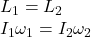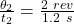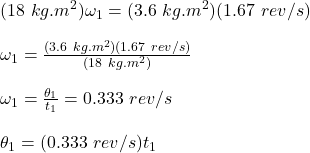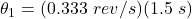A diver comes off a board with arms straight up and legs straight down, giving her a moment of inertia about her rotation axis of 18kg⋅m2. S

Question

A diver comes off a board with arms straight up and legs straight down, giving her a moment of inertia about her rotation axis of 18kg⋅m2. She then tucks into a small ball, decreasing this moment of inertia to 3.6kg⋅m2. While tucked, she makes two complete revolutions in 1.2s.

Required:
If she hadn’t tucked at all, how many revolutions would she have made in the 1.5 s from board to water?

in progress 0
5 months 2021-08-11T11:07:58+00:00 1 Answers 106 views 0

θ₁ = 0.5 revolution

Explanation:

We will use the conservation of angular momentum as follows:where,

I₁ = initial moment of inertia = 18 kg.m²

I₂ = Final moment of inertia = 3.6 kg.m²

ω₁ = initial angular velocity = ?

ω₂ = Final Angular velocity == 1.67 rev/s

Therefore,where,

θ₁ = revolutions if she had not tucked at all = ?

t₁ = time = 1.5 s

Therefore,θ₁ = 0.5 revolution